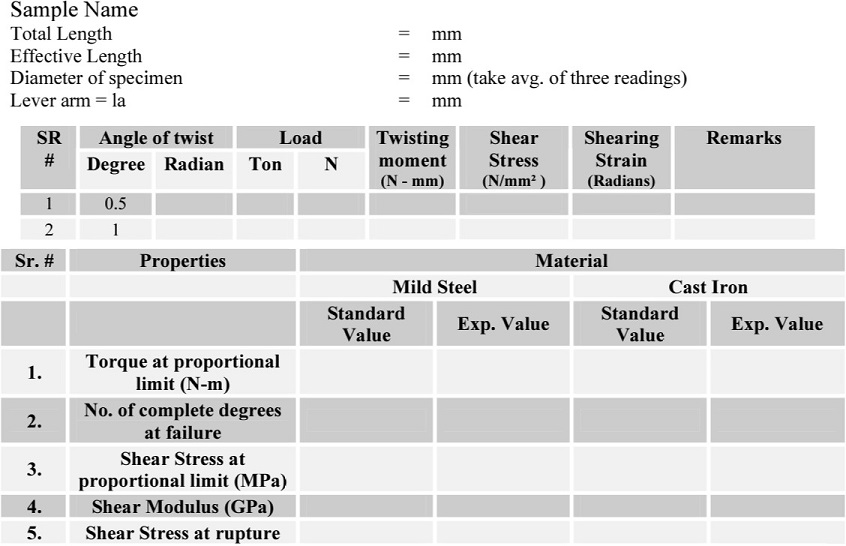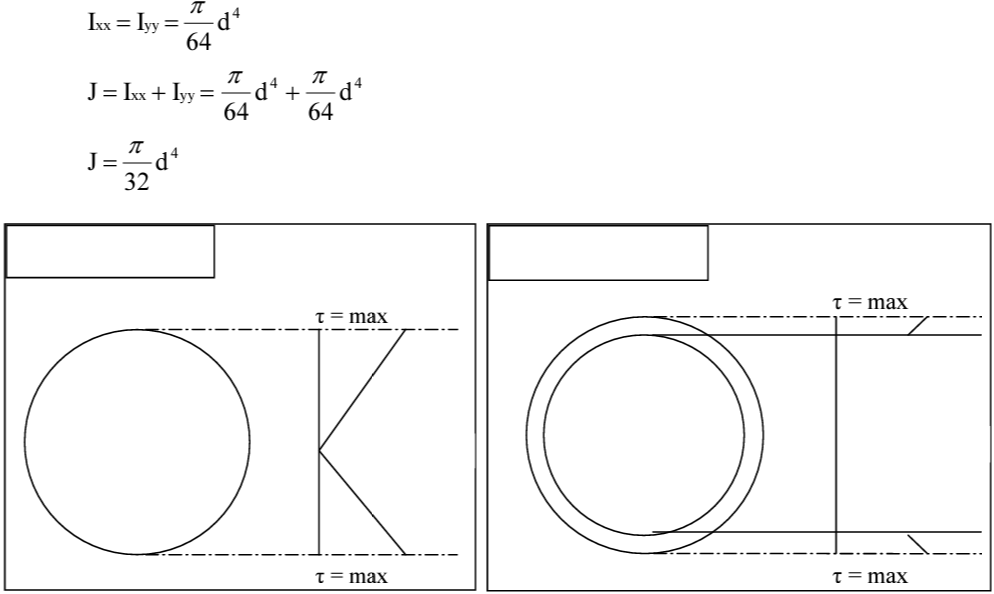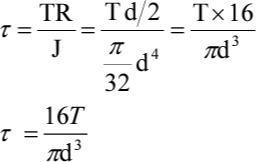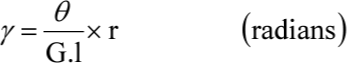Feb 26, 2016

Feb 26, 2016

Feb 26, 2016

# Torsion Test on Mild Steel and Cast Iron - Lab Report

To perform Torsion Test on

1. Mild steel specimen
2. Cast iron specimen

## Purpose:

1. To study the shear stress ~ shear strain behavior of the material.
2. To study the failure pattern of these materials in torsion.
3. To determine the mechanical properties, e.g, Modulus of elasticity, Modulus of rigidity, Shear strength, shear strain and ductility in torsion.

## Apparatus:

1. 10 Ton Buckton Universal Testing Machine
2. Vernier caliper
3. Steel Rule

## Procedure of the Test:

1. Note the dimensions and draw the shape of the specimen.
( Note effective length, total length, dia meter etc.)
2. Fix specimen into 10 Ton Buckton UTM.
3. Use twist control method (other one is torsional strain control method)
4. To apply the twist to the sample, rotate the handle counter clock wise for required degree of twist. Machine’s one complete cycle will give 4° of twist.
5. Balance arm of the machine will get disturbed again. Try to balance it with the help of concerned handle and note down the value of balancing load.
6. Repeat the same procedure with increasing value of twist until the member fails.
Lever arm = 50.8mm
Torque = lever arm x load 16T7. Examine the failure pattern of the specimen and draw sketch after failure.(same for cast iron )

## Observations and Calculations:## Related Theory:

### 1. Torque:

Twisting effect of couple or force is called as torque. It is denoted by double head arrow.

### 2. Torsion:

Torque applied in a plane perpendicular to the longitudinal axis of a member is called as
torsion.

### 3. Difference between Torque and Moment:### 4. Twisting Moment:

It is the summation of torque either left or right of the section.

### 5. Bending Theory:### 6. Torsion Theory:

#### Assumptions:

1. Material is homogeneous.
2. Circular section remains circular and do not warp.
3. A plane section of a material perpendicular to its longitudinal axis remain plane and do not warp after the torque is applied.
4. Shaft is loaded by a couple or torque in a plane perpendicular to the longitudinal axis of the plane.
5. Shear stress is proportional to shear strain, it means that Hook’s Law is applicable.
6. In circular shafts subjected to torque shearing strain varies linearly.Where,

t, Shearing stress in MPa
r, Radius of the shaft in mm.
T, Twisting moment.
J, Polar moment of inertia.
G, Modulus of rigidity.
θ Angle of twist.
L, Length of the specimen / Shaft

### 7. Polar moment of inertia:

The geometric rigidity of the X-sec is termed as polar moment of inertia. It is the resistance against twisting, summation of 2 moment of area about x-axis.

#### Circular Section:#### For Hollow Shaft:#### Torsional Rigidity / Modulus of Rigidity / Modulus of Elasticity in Shear:"When material is subjected to pure twist loading, the slope of shear stress verses shear strain curve is termed as modulus of rigidity ( modulus of elasticity in shear, torsional rigidity)### 9. Poison’s Ratio:

The ratio of lateral strain to longitudinal strain when material is subjected to axial loading and always less than 1.### 10. Relation between yield strength in tension to torsion for mild steel:

The relationship between yield stress in simple tension and that in pure shear can be found from VON MISES for a yield criteria.### 11. Torsional Strength:

• It is the ultimate strength of a material subjected to a torsional loading.
• It is maximum torsional stress that a material sustains before rupture.
• It is similar to the tensile strength.

### 12. Torsional Deformation:

Angular displacement of specimen caused by specified torque in torsion test. It is equal to angle of twist in radians divided by gauge length or effective length.

### 13. Torsional Strain, y:

Strain corresponding to specified torque in torsion test. It is equal to torsional deformation multiplied by the radius of the shaft. It's units are radians.### 14. Torsional Stress, T:

Shear stress developed in a material subjected to a specified torque in torsion test for a circular shaft. It can be calculated using the expression.### 15. Expected failure for Mild Steel and Cast Iron Specimens and reasoning:

Fracture in torsion for ductile materials generally occur in the plane of maximum shear stress perpendicular to the axis of bar where as for the brittle material failure occurs along 45° hilux to the axis of bar due to tensile stress across that plane.